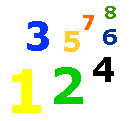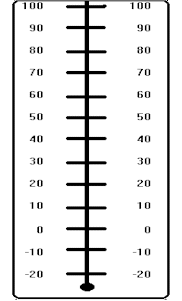# Telling Temperature

Telling Temperature Lesson & Worksheet - My Schoolhouse - Online Learning

Look at the thermometer.

Find 70 degrees.  70 degrees is just right for most people.

Find 100 degrees.  100 degrees is a very, very hot day.

Find 32 degrees.  When it is 32 degrees, water will freeze.

Can you find 0 (zero) on the thermometer?  Do you see there are numbers below the 0?  In some places in the winter it gets very cold.  It goes below zero!1. Is 20 degrees above or below 30 degrees?

above
below

2. Is 32 degrees above or below 30 degrees?

above
below

3. Will it rain or snow if the temperature is 28 degrees?

rain
snow

4. Can you swim outdoors if the temperature is 90 degrees?

yes
no

5. Is -20 degrees above or below 0 (zero)?

above
below

6. The temperature in school is 70 degrees.  Is this a comfortable temperature for most people?

yes
no

7. Will an ice cream cone melt fast if the temperature is 90 degrees?

yes
no

8. Will a pond freeze if the temperature is 0 (zero)?

yes
no

9. Is 0 (zero) above or below -10 degrees?

above
below

10. Which temperature is hotter?

80 degrees
40 degrees

11. Which temperature is colder?

10 degrees
-10 degrees

12. Which temperature is colder than 0 (zero)?

32 degrees
-20 degrees